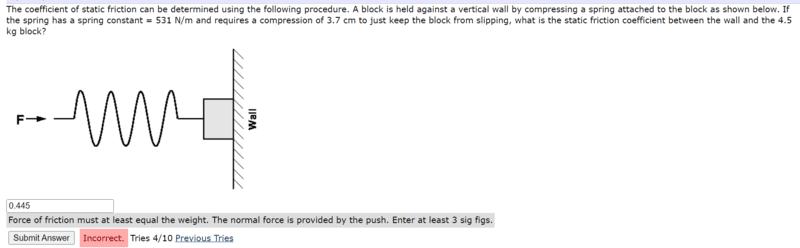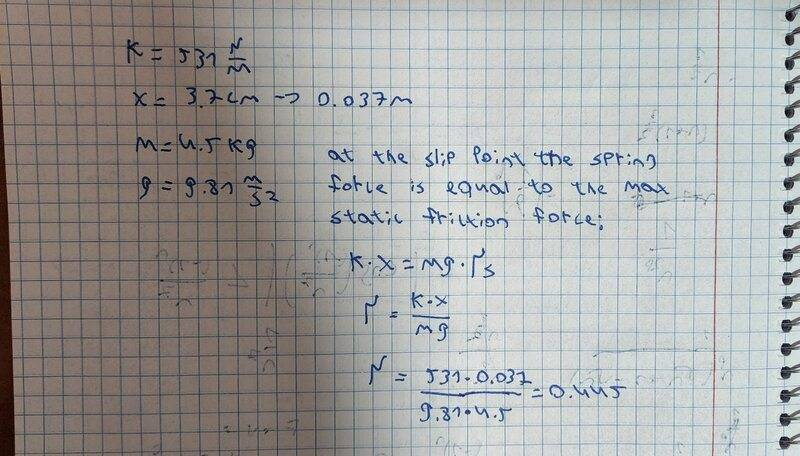# Blockage Held by Spring

• yesmale4

#### yesmale4

Homework Statement
The coefficient of static friction can be determined using the following procedure. A block is held against a vertical wall by compressing a spring attached to the block as shown below. If the spring has a spring constant = 531 N/m and requires a compression of 3.7 cm to just keep the block from slipping, what is the static friction coefficient between the wall and the 4.5 kg block?
Relevant Equations
f=x*ki would like to understand why my answer is incorrect and how i need to solve this problem

Looks to me like you've got things upside down. Given that spring compression, what's the force pushing on the block? How does that relate to the friction?

Based on this relation,

(fs)max = μs N

the value of μs must be greater than 1 in this case, as (fs)max > N

Looks to me like you've got things upside down. Given that spring compression, what's the force pushing on the block? How does that relate to the friction?
Fspring= x*k = 0.037*531
i don't see how the friction is relate because the only force is Fs-mg=0

Based on this relation,

(fs)max = μs N

the value of μs must be greater than 1 in this case, as (fs)max > N
i understand so how i need to change my calculations to get to the right answer

Fspring= x*k = 0.037*531
That's correct.
i don't see how the friction is relate because the only force is Fs-mg=0
If by Fs you mean the spring force, that is incorrect. The only vertical forces acting on the block are gravity and friction (not the spring force). The spring force is like the normal force (N). To get the friction you multiply by μ. Then you'll have μN - mg = 0. Solve for μ. (You had it upside down.)

•Lnewqban
i understand so how i need to change my calculations to get to the right answer
Perhaps if you turn the picture 90 degrees clockwise, you could see the more traditional problem.
Please, refer to post #6 above.

If by Fs you mean the spring force
More likely it stands for the static friction force.
If so, @yesmale4 , what are the relationships between:
##F_{spring}, F_s, N##?